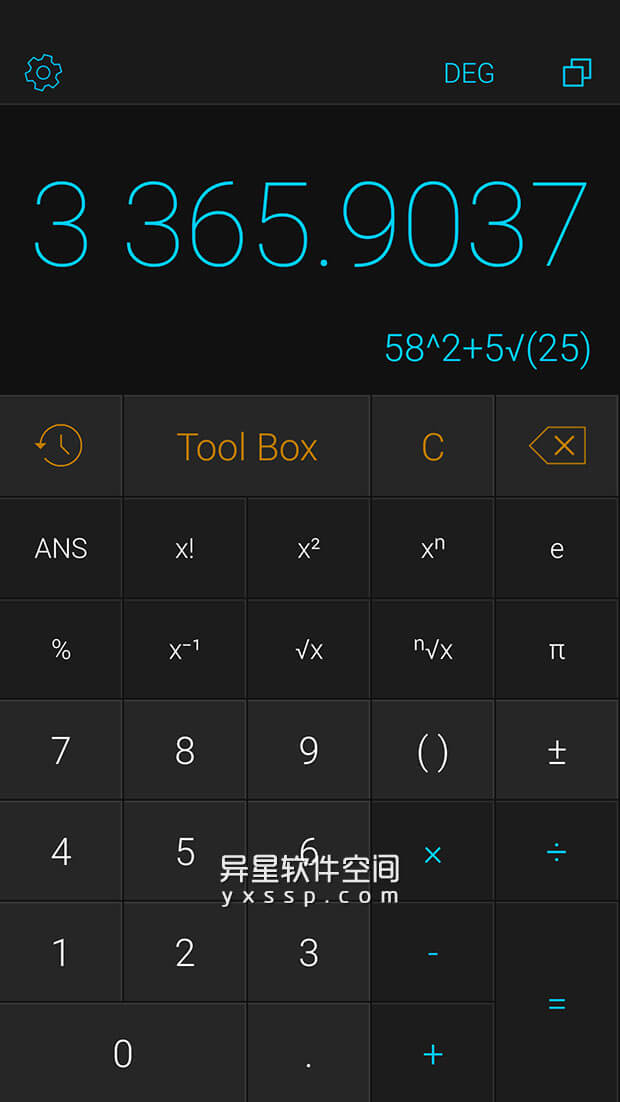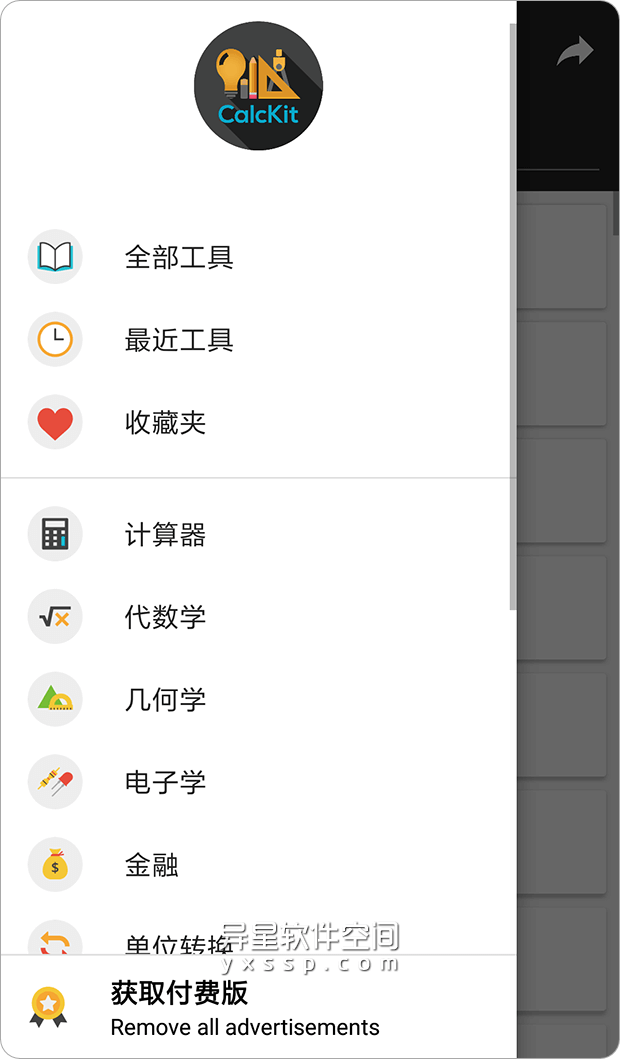# CalcKit: All in One Calculator「CalcKit：多合一计算器」v3.0.6 for Android 解锁高级版 —— 一款强大的多合一计算器合集，适用于大多数专业

0CalcKit: All in One Calculator「CalcKit：多合一计算器」是一款拥有超过150种计算器和单位转换器，内置高度定制的科学计算器，甚至能让你创建你自己的计算器和转换器，CalcKit 应该是一款您梦寐以求的多合一计算器。### CalcKit精彩功能

• 超过150种计算器和单位转换器
• 高度定制的科学 & RPN(逆波兰表示法)计算器
• 悬浮计算器小部件
• 完全可编辑的计算器历史
• 日间&夜间计算器主题
• 支持160个币种的货币兑换，可离线使用
• 数学表达式支持输入形式
• 即时结果
• 使用多达25个变量创建自定义工具
• 集成搜索
• 集成笔记本
• 添加快捷方式到你的主屏幕以快速访问

### 计算器和转换器列表

• 线性、二次方程式和三次方程
• 2×2和3×3方程组

• 两点间距
• 三角形面积
• 圆形和球体的方程式

• 素数检查
• 比例计算器
• 分数简化
• 小数转分数
• 素因子分解
• 最大公因子/最小公倍数
• 组合/排列

• 行列式、倒置、移项
• A × B、A + B、A – B

• 欧姆定律
• 功率三角形
• Y – Δ 变换
• 稳压器
• NE555定时器
• 电抗
• 滤波器
• 电池寿命计算器
• 导线电阻率
• 变压比
• 模拟 – 数字转换
• 运算放大器
• LED电阻计算器
• 频率计算器
• 串联/并联元件
• 色码电感器
• 色码电阻器
• 分压器

• 货币兑换
• 小费计算器
• 增值税/税金计算器

• 常用
• 数字
• 罗马数字
• 词头
• 角度
• 面积
• 烹饪
• 数据存储
• 耗油量
• 质量/重量
• 长度/距离
• 温度
• 时间
• 速度
• 压力
• 功率
• 体积
• 力
• 能量/功
• 鞋码

• 电荷
• 电流
• 电导
• 电导率
• 电场强度
• 电势
• 电阻
• 电阻率
• 静电电容
• 电感
• 线性电荷密度
• 线性电流密度
• 表面电荷密度
• 表面电流密度
• 体电荷密度

• 加速度
• 加速度 – 角度
• 气流
• 密度
• 惯性矩
• 比容积
• 转矩
• 速度 – 角度

• 浓度 – 摩尔
• 浓度 – 溶液
• 流量
• 流量 – 质量
• 流量 – 摩尔
• 质通量密度
• 渗透性
• 表面张力
• 黏度 – 动态
• 黏度 – 运动

• 燃料效率 – 质量
• 燃料效率 – 体积
• 热密度
• 热流密度
• 传热系数
• 比热容
• 温度间距
• 热导率
• 热膨胀
• 热阻

• 照明度
• 亮度
• 光度

• 磁场强度
• 磁通量
• 磁通密度
• 磁通势

• 辐射
• 辐射 – 吸收剂量
• 辐射 – 放射性
• 辐射 – 照射量

• 频率
• 图片分辨率
• 步速
• 像素密度
• 声音
• 字体排版
• 体积 – 干性
• 体积 – 木材

### 更新内容如下：

• 总体设计改进
• 简化的ToolBox导航
• 改进的搜索
• 用于日期/时间计算的新工具
• 计算器的全键盘布局
• 改进的浮动计算器
• 错误修复及更多…

### 关于版本：

• 高级功能已解锁；
• 优化的图形和Zipalign；
• 禁用/删除不需要的权限+接收者和服务；
• Analytics（分析）/ Crashlytics（崩溃）已禁用。
• 运行系统要求：Android 4.2+